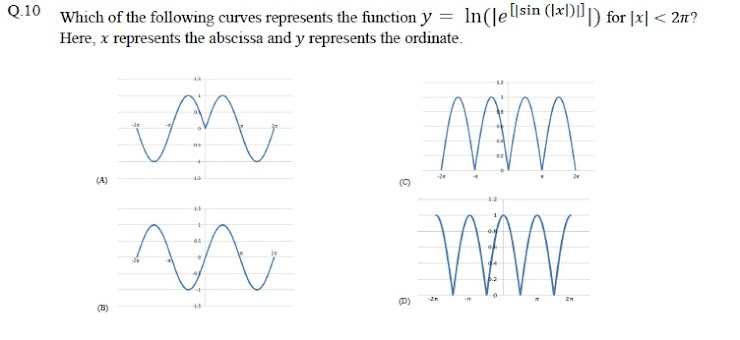GATE(Biotechnology)Mock Test
Q1.The volume of a sphere of diameter 1 unit is ________ than the volume of a cube of side 1 unit. *
Q.2 The unruly crowd demanded that the accused be _____________ without trial. *
Q3 Choose the statement(s) where the underlined word is used correctly: (i) A prone is a dried plum. (ii) He was lying prone on the floor. (iii) People who eat a lot of fat are prone to heart disease. *
Q 4 Fact: If it rains, then the field is wet. Read the following statements: (i) It rains (ii) The field is not wet (iii) The field is wet (iv) It did not rainWhich one of the options given below is NOT logically possible, based on the given fact? *
Q5. A window is made up of a square portion and an equilateral triangle portion above it. The base ofthe triangular portion coincides with the upper side of the square. If the perimeter of the window is6 m, the area of the window in m2 is ___________. *
Q 6 Students taking an exam are divided into two groups, P and Q such that each group has the samenumber of students. The performance of each of the students in a test was evaluated out of 200marks. It was observed that the mean of group P was 105, while that of group Q was 85. Thestandard deviation of group P was 25, while that of group Q was 5. Assuming that the marks weredistributed on a normal distribution, which of the following statements will have the highestprobability of being TRUE? *
Q7 A smart city integrates all modes of transport, uses clean energy and promotes sustainable use ofresources. It also uses technology to ensure safety and security of the city, something which criticsargue, will lead to a surveillance state.Which of the following can be logically inferred from the above paragraph?(i) All smart cities encourage the formation of surveillance states.(ii) Surveillance is an integral part of a smart city.(iii) Sustainability and surveillance go hand in hand in a smart city.(iv) There is a perception that smart cities promote surveillance. *
Q.8. Find the missing sequence in the letter series.B, FH, LNP, _ _ _ _. *
Q9.The binary operation □ is defined as a □ b = ab+(a+b), where a and b are any two real numbers.The value of the identity element of this operation, defined as the number x such that a □ x = a, for any a, is .(A) 0 (B) 1 (C) 2 (D) 10
Q10 *